# Blood Relation MCQ - 4

## 20 Questions MCQ Test Reasoning Aptitude for Competitive Examinations | Blood Relation MCQ - 4

Description
Attempt Blood Relation MCQ - 4 | 20 questions in 15 minutes | Mock test for Banking Exams preparation | Free important questions MCQ to study Reasoning Aptitude for Competitive Examinations for Banking Exams Exam | Download free PDF with solutions
QUESTION: 1

Solution:
QUESTION: 2

Solution:
QUESTION: 3

### Study the following information carefully to answer the given questions X @ Y means x is wife of y X © Y means x is sister of y X % Y means x is son of y X # Y means x is mother of y X \$ Y means x is father of y Q.  Which of the following expressions represents M is brother of P ?

Solution:
QUESTION: 4

Study the following information carefully to answer the given questions
X @ Y means x is wife of y
X © Y means x is sister of y
X % Y means x is son of y
X # Y means x is mother of y
X \$ Y means x is father of y

Q.

What will come in the place of ? if A is maternal grandfather of B
E @ A \$ C ?  D © B

Solution:
QUESTION: 5

Study the following information carefully to answer the given questions
P × Q means P is father of Q
P ÷ Q means P is daughter of Q
P + Q means P is sister of Q
P – Q means P is husband of Q
In M ÷ N × O – P, How O related to M ?

Solution:
QUESTION: 6

Study the following information carefully to answer the given questions
X @ Y means x is wife of y
X © Y means x is sister of y
X % Y means x is son of y
X # Y means x is mother of y
X \$ Y means x is father of y
How is J related to K in the given expression : K % H © I @ J \$ O

Q.

What will come in the place of ? if A is maternal grandfather of B
E @ A \$ C ?  D © B

Solution:
QUESTION: 7

Study the following information carefully to answer the given questions
P × Q means P is father of Q
P ÷ Q means P is daughter of Q
P + Q means P is sister of Q
P – Q means P is husband of Q
In M ÷ N × O – P, How O related to M ?

Solution:
QUESTION: 8

Study the following information carefully to answer the given questions
P × Q means P is father of Q
P ÷ Q means P is daughter of Q
P + Q means P is sister of Q
P – Q means P is husband of Q
In M ÷ N × O – P, How O related to M ?

Q.

In G×T+Q÷M, how is M related to G ?

Solution:

Explanation : G×T+Q÷M

G(male) is father of T

T(female) is sister of Q

Q(female) is daughter of M

From the equations, we came to know, M is the wife of G.

QUESTION: 9

Introducing a woman, Nishanthi said, ‘She is the daughter-in-law of the grandmother  of my father’s only son.” How is the woman related to Nishanthi ?

Solution:
QUESTION: 10

A introduced B as the son of the son of the father of her uncle. How is A related to B.

Solution: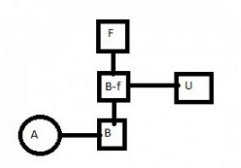QUESTION: 11

Rakesh introduced priya as the sister of the father of my mother’s son . How is priya related to rakesh ?

Solution: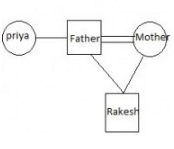QUESTION: 12

In a family of 6 members P,Q,R,S,T and U, there are two married couples. P and U are married to each other. P and U have 2 children T and Q. Q a male is married to R and has one daughter S.
How is R related to P.

Solution: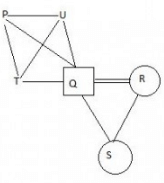QUESTION: 13

In a family of 6 members P,Q,R,S,T and U, there are two married couples. P and U are married to each other. P and U have 2 children T and Q. Q a male is married to R and has one daughter S.

Q. What is the relation between T and R.

Solution:
QUESTION: 14

In a family of 6 members P,Q,R,S,T and U, there are two married couples. P and U are married to each other. P and U have 2 children T and Q. Q a male is married to R and has one daughter S.
How is R related to P.

Q.

How is S related to T.

Solution:
QUESTION: 15

Directions for Q 15 - 18

In a family of 6 members P,Q,R,S,T and U, there are two married couples. P and U are married to each other. P and U have 2 children T and Q. Q a male is married to R and has one daughter S.
How is R related to P.

Q.

A + B means ‘A is the father of B’
A- B means ‘A is the husband of B’
A*B means ‘A is the sister of B’
A%B means ‘A is the brother of B’
A@B means ‘A is the mother of B’
What is the relation of A with D in ‘A+B*C-D’?

Solution:
QUESTION: 16

A and B are brothers. C and D are sisters. A's son is D's brother. How is B related to C ?

Solution:

C and D are the daughters of A and B is the uncle of C.

QUESTION: 17

What should be placed in place of question mark in ‘A * D? P – R ‘so that R becomes daughter in law of D.

Solution:
QUESTION: 18

How many male members are there in the expression P + Q – R * S @ T.

Solution: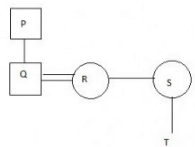QUESTION: 19

M is daughter of N and sister of L. K is daughter of L and granddaughter of G. F is sister-in-law of M. L is only sibling of M.
How is K related to N ?

Solution: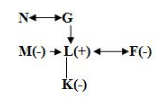QUESTION: 20

M is daughter of N and sister of L. K is daughter of L and granddaughter of G. F is sister-in-law of M. L is only sibling of M.
How is K related to N ?

Q.

How is F related to G ?

Solution:Use Code STAYHOME200 and get INR 200 additional OFF Use Coupon Code